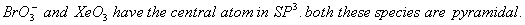Monday 08th August 2022

# AIPMT 2007 Chemistry Screening

Q. 1. With which of the following configuration an atom has the lowest ionization enthalpy?

1.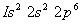2.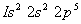3.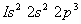4.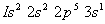Sol.represents the excited state of a Neon atom. The energy needed to knock off an electron from the excited state of neon must be least. Correct choice is: (4)

Q. 2. An element, X has the following isotopic composition; 200X:90% ; 199X:8.0% ; 202X:2.0%
The weighted average atomic mass of the naturally occurring element X is closest to :

1. 199 amu
2. 200 amu
3. 201 amu
4. 202 amu

Sol. The weighted average atomic mass of element (X) = 0.9 (200) + 0.08 (199) + 0.02 (202) = 180 + 15.92 + 4.04 =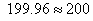Correct choice is: (2)

Q. 3. Concentrated aqueous sulphuric acid is 98% H 2SO 4 by mass and has a density of 1.80 g. mL-1 . Volume of acid required to make one litre of 0.1 M H2SO4 is,

1. 5.55 mL
2. 11.10 mL
3. 16.65 mL
4. 22.20 mL

Sol.  Density = 1.80 g/ml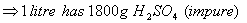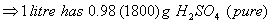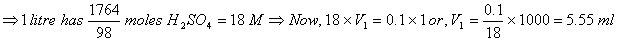Correct choice: (1)

Q.4. Consider the following sets of quantum numbers:

 n I M s (a) 3 0 0 + ½ (b) 2 2 1 + ½ (c) 4 3 -2 - ½ (d) 1 0 -1 - ½ (e) 3 2 3 +1/2

Which of the following sets of quantum number is not possible

1. a and c
2. b, c and d
3. a, b, c and d
4. b, d and e

Sol. Choice (b), (d) and (e) are incorrect. Remember that value of ‘l’ ranges from (0) to (n – 1) and values of ‘m’ range from (–l) to (+l). Correct choice: (4)

Q. 5. The number of moles of KMnO4 that will be needed to react with one mole of sulphite ion in acidic solution is:

1. 1
2.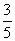3.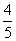4.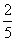Sol.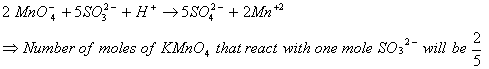Correct choice : (4)

Q. 6. In a first-order reaction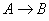, if k is rate constant and initial concentration of the reactant A is 0.5 M then the half-life is :

1.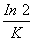2.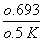3.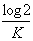4.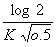Sol.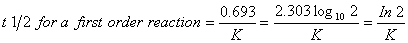Correct choice: (1)

Q. 7. The reaction of hydrogen and iodine monochloride is given as: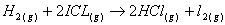This reaction is of first order with respect to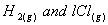, following mechanisms were proposed :

Mechanism A :Mechanism B :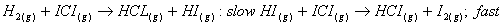Which of the above mechanism (s) can be consistent with the given information about the reaction

1. A only
2. B only
3. 1 and 2 both
4. Neither 1 nor 2

Sol. The rate law is invariably determined from the slowest step of the mechanism. Therefore mechanism (B) is consistent with the data given for order of reaction. Correct choice: (2)

Q. 8. If 60% of a first order reaction was completed in 60 minutes, 50% of the same reaction would be completed in approximately :

1. 40 minutes
2. 50 minutes
3. 45 minutes
4. 60 minutes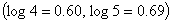Sol.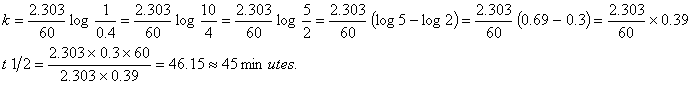Correct choice: (3)

Q. 9. The equilibrium constant of the reaction :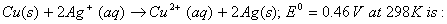1.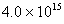2.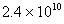3.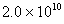4.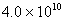Sol.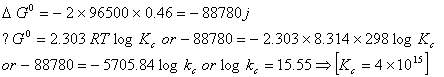Correct choice: (1)

Q. 10. 0.5 molal aqueous solution of a weak acid (HX) is 20% ionized. If Kf for water is 1.86 K kg mol sup-1 , the lowering in freezing point of the solution is

1. – 0.56 K
2. – 1.12 K
3. 0.56 K
4. 1.12 K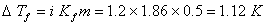Correct choice: (4)

Q. 11.The efficiency of a fuel cell is given by

1.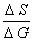2.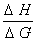3.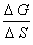4.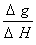Sol. Efficiency of a fuel cell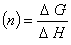Correct choice: (4)

Q. 12. Consider the following reactions:

1.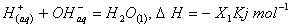2.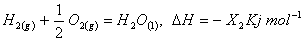3.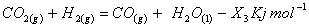4.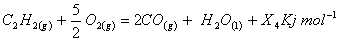Enthalpy of formation of H2O(l) is

1.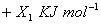2.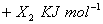3.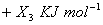4.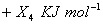Sol. Heat of formation of H2O(l) = -X2 kJ / mol . Correct choice: (2)

Q. 13. Given that bond energies of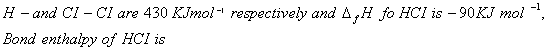1.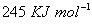2.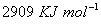3.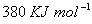4.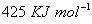Sol.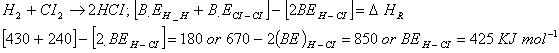Correct choice: (4)

Q. 14. The Langmuir adsorption isotherm is deduced using the assumption

1. The adsorbed molecules interact with each other
2. The adsorption takes place in multilayers
3. The adsorption sites are equivalent in their ability to adsorb the particles
4. The heat of adsorption varies with coverage

Sol. angmuir adsorption has the following postulates

1. The isotherm is devised for adsorption equilibrium i.e., when rate of adsorption = rate of desorption
2. Adsorption at all sites is equivalent
3. Adsorption at a site is unaffected by adsorption at neighboring sites.

Correct choice: (3)

Q. 15. The following equilibrium constants are given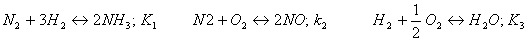The equilibrium constant for the oxidation of NH3 by oxygen to give NO is

1.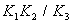2.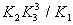3.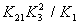4.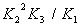Sol.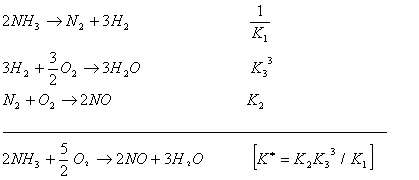Correct choice: (2)

Q. 16. Caulate the pOH of a solution at 250C that contains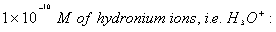1. 1.000
2. 7.000
3. 4.000
4. 9.000

Sol.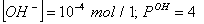Correct choice : (3)

Q. 17. Aweak acid,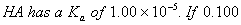mol of this acid is dissolved in one litre of water, the percentage of acid dissociated at equilibrium is closest to

1. 0.100%
2. 99.0%
3. 1.00%
4. 99.9%

Sol. We know that,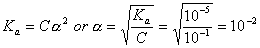Correct choice: (3)

Q. 18. The fraction of total volume occupied by the atoms present in a simple cube is

1.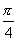2.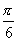3.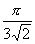4.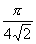Sol. Packing fraction for a simple cube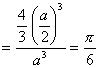Correct choice: (2)

Q. 19. Identify the correct order of the size of the following:

1.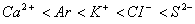2.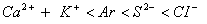3.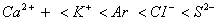4.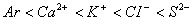Sol. Anions have the largest sizes followed by neutral atoms and then cations for a respective period. So, the correct order should beCorrect choice: (3)

Q.20. In which of the following pairs, the two species are iso-structural?

1.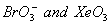2.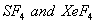3.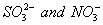4.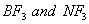Sol. Both -